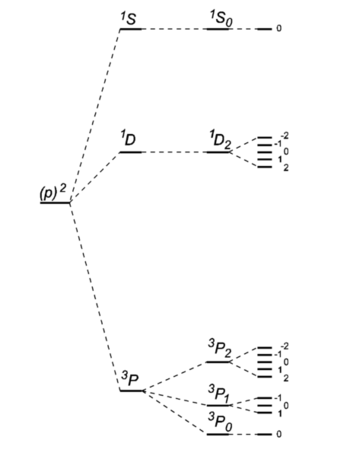# Hund's rules

In atomic spectroscopy, Hund's rules predict the order of atomic energy levels with quantum numbers L (orbital), S (spin) and J (orbital plus spin). The rules are called after Friedrich Hund who formulated them in 1925.

## LS coupling

A group of atomic energy levels, obtained by Russell-Saunders coupling, is concisely indicated by a term symbol. As discussed in the article Russell-Saunders coupling, closed shells and closed subshells have L = S = 0 and hence can be ignored in the coupling. A term (also known as multiplet) is a set of simultaneous eigenfunctions of L2 (total orbital angular momentum squared) and S2 (total spin angular momentum squared) with given quantum numbers L and S, respectively. That is, the respective eigenvalues are L(L+1)2 and S(S+1)2.

If there is no spin-orbit coupling, the functions of one term (fixed L and S) are (2L+1)×(2S+1)-fold degenerate (have the same energy). If there is weak spin-orbit coupling it is useful to diagonalize the matrix of the corresponding spin-orbit operator within the L-S basis [consisting of the (2L+1)×(2S+1) functions of the term] in the spirit of first-order perturbation theory. This lifts partially the degeneracy and introduces the new conserved quantum number J, with |L-S| ≤ JL+S, that labels a (2J+1)-fold degenerate energy level.Level scheme of the carbon atom$(1s)^2(2s)^2(2p)^2$. Drawing is not on scale. On the left the energy without any two-particle interaction. Then three-fold energy splitting after switching on electrostatic electron-electron interaction (L and S good quantum numbers). Then splittings after switching on first-order spin-orbit coupling (J good quantum number). Finally on the right Zeeman splittings in an external magnetic field.

## Formulation of the rules

Hund's rules are:

1. Of the Russell-Saunders states arising from a given electron configuration those with the largest spin quantum number S lie lowest, those with the next largest next, and so on; in other words, the states with largest spin multiplicity are the most stable.
2. Of the group of terms with a given value of S, that with the largest value of L lies lowest.
3. Of the states with given values of S and L in an electronic configuration consisting of less than half the electrons in a closed subshell, the state with the smallest value of J is usually the most stable, and for a configuration consisting of more than half the electrons in a closed subshell the state with largest J is the most stable.

The levels of the second sort, largest J most stable, can be seen as arising from holes in a closed subshell.

## Examples

• The ground state carbon atom, (1s)2(2s)2(2p)2, gives by Russell-Saunders coupling a set of energy levels labeled by term symbols. Hund's rules predict the following order of the energies:
3P0 < 3P1 < 3P2 < 1D2 < 1S0.
• The ground state oxygen atom, (1s)2(2s)2(2p)4, (a two-hole state) gives by Russell-Saunders coupling a set of energy levels labeled by term symbols. Hund's rules predict the following order of the energies:
3P2 < 3P1 < 3P0 < 1D2 < 1S0.